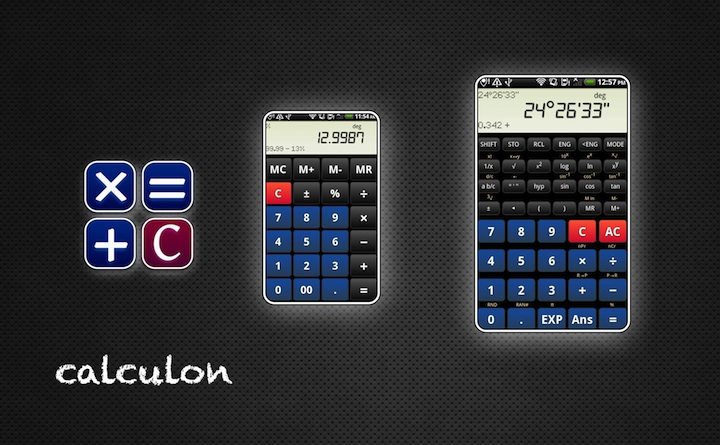# Calculon

Calculon is our awesome, super fast easy-to-use full-fledged calculator.

He is the cloned brother of droid calc and runs on Blackberry PlayBooks OS2.0+.

The app shares many features with old school calculator: it comes with decimal, sexagesimal, exponential and fractional displays. It performs arithmetic, trigonometric and logarithmic functions. It knows about permutations, combinations and coordinate conversion. And you have 7 memory cells to store your results.

Choose the way you want to input arithmetic expressions. With input method "infix" you can type "sin 45". You would type "45 sin" if you prefer the old-style input method.

• It's super fast and has an awesome responsiveness.
• Swipe the display and switch seamlessly between 4 different scientific skins and one basic skin.
• The layout adapts itself to different device configurations.

### Really simple input

Example: 10 + 2×6 - 15 ÷ (1 + 2) = 17

input display
1 0 + 10. +
2 × 6 - 22. -
1 5 ÷ ( ( 0.
1 + 2 ) 3.
= = 17.

The following examples use a shorter notation.

Instead of 10.25 you would type 10.25.

Calculon accepts algebraic (infix) and old-style input methods. You can set the input method to be used on the settings menu.

An example with algebraic (infix) input method: √2 ÷ cos -45° = 2

2÷cos45+/-== 2.

The same example with old-style input method: √2 ÷ cos -45° = 2

2÷45+/-cos== 2.

### Number input

Category Example Input
rational numbers 0.3 .3 or 0.3
negative numbers -4.7 4.7+/-
exponential notation 3×102 (=300) 3EXP2
-5.2×103 (=-5200) 5.2+/-EXP3
-8.3×10-4 (=-0.00083) 8.3+/-EXP4+/-
pi π (=3.141592653...) EXP
fractions 2/3 (=0.666666...) 2a b/c3
5 1/4 (=5.25) 5a b/c1a b/c4
degrees 5° 20' 30" (=5.341666...) 5° ' "20° ' "30° ' "

### Functions 1

argumentfunction

For these functions you enter the argument followed by the function key.

Function Example Input Display
1/x Reciprocal 2-1 = 0.5 21/x= 0.5
x2 Square 32 = 9 3x2= 9.
x! Factorial 3! = 6 3SHIFTx!= 6.

### Functions 2

Calculon comes with 2 input methods: algebraic (infix) and old-style. You can set the input method to be used on the settings menu.

### algebraic (infix) input method

functionargument

Modern scientific calculators often use algebraic (infix) input method.

For these functions you press the function key followed by the argument if the input method is set to algebraic (infix).

Function Example Display
Square root √9 = 3
9=
3.
3 Cube root 3√27 = 3
SHIFT327=
3.
log Common logarithm log 100 = 2
log100=
2.
10x Powers of 10 102 = 100
SHIFT10x2=
100.
ln Natural logarithm ln 10 = 2.302585093
ln10=
2.302585093
ex Powers of e e2.302585093 = 10
SHIFTex2.302585093=
10.
sin
cos
tan
Sine, cosine, tangent sin 30° = 0.5
sin30=
0.5
sin-1
cos-1
tan-1
Arc
sine, cosine, tangent
asin 0.5 = 30°
SHIFTsin-1.5=
30.
hypsin
hypcos
hyptan
Hyperbolic
sine, cosine, tangent
sinh 5 = 74.20321058
hypsin5=
74.20321058
hypsin-1
hypcos-1
hyptan-1
Inverse hyperbolic
sine, cosine, tangent
sinh-1 74.20321058 = 5
SHIFThypsin-174.20321058=
5.

### old-style input method

argument function

Older, more basic calculators often use this input method.

For these functions you enter the argument first and then press the function key if the old-style input method is set.

function example display
Square root √9 = 3
9=
3.
3 Cube root 3√27 = 3
27SHIFT3=
3.
log Common logarithm log 100 = 2
100log=
2.
10x Powers of 10 102 = 100
2SHIFT10x=
100.
ln Natural logarithm ln 10 = 2.302585093
10ln=
2.302585093
ex Powers of e e2.302585093 = 10
2.302585093SHIFTex=
10.
sin
cos
tan
Sine, cosine, tangent sin 30° = 0.5
30sin=
0.5
sin-1
cos-1
tan-1
Arc
sine, cosine, tangent
asin 0.5 = 30°
.5SHIFTsin-1=
30.
hypsin
hypcos
hyptan
Hyperbolic
sine, cosine, tangent
sinh 5 = 74.20321058
5hypsin=
74.20321058
hypsin-1
hypcos-1
hyptan-1
Inverse hyperbolic
sine, cosine, tangent
sinh-1 74.20321058 = 5
74.20321058SHIFThypsin-1=
5.

### Functions with two arguments

argument 1functionargument 2

function example display
xy Powers 23 = 8
2xy3=
8.
x Roots 7√128 = 2
7SHIFTx128=
2.
nPr Permutations 6 nPr 3 = 120
6SHIFTnPr3=
120.
nCr Combinations 6 nCr 3 = 20
6SHIFTnCr3=
20.
R→P Rectangular to polar
coordinate conversion
x=3, y=4: 3 RP 4 = 5 (r), 53.13010235 (θ)
3SHIFTR→P4=
r = 5.
SHIFTx ↔ y θ = 53.13010235
P→R Polar to rectangular
coordinate conversion
r=2, θ=60: 2 PR 60 = 1 (x), 1.732050808 (y)
2SHIFTP→R60=
x = 1.
SHIFTx ↔ y y = 1.732050808

### Percentage calculations

You can set the kind of percentage calculation to be used on the settings page (gear in top right corner).

Modern scientific calculators often use this kind of percentage calculation. The advanced percentage calculation answers several questions easily.

19% of 120 = 22.8

120×19SHIFT% 22.8

Percentage of 80 represented by 25 = 31.25%

25÷80SHIFT% 31.25

19% add-on of 120 = 142.8

120×19SHIFT%+ 142.8

30% discount of 1000 = 700

1000×30SHIFT%- 700.

20 apples are added to a basket with 50 apples. What is the percentage of the new number of apples to the initial number of apples?: 140%

20+50SHIFT% 140.

If you got 50 EUR last week and 80 EUR this week, what is the percent increase?: 60%

80-50SHIFT% 60.

### Percentage calculations - simple

Basic calculators come often with this kind of percentage calculation.

example input display
200 + 19% = 238 200+19SHIFT%= 238.
238 - 19% = 192.78 238-19SHIFT%= 192.78
200 ÷ 50% = 2 200÷50SHIFT%= 2.
200 × 50% = 20000 200×50SHIFT%= 20000.

With your input we can further improve our services.

comment

email

Used for a reply message. The content of this field is kept private and will not be shown publicly.

# History

## Version 1.2

BlackBerry App World 2012-02-27

• initial version.